# Event-related averaging and MEG planar gradient

## Introduction

This tutorial works on the MEG-language dataset, you can click here for details on the dataset. This tutorial is a continuation from the preprocessing tutorials. We will begin by repeating some code used to select the trials and preprocess the data as described in the earlier tutorials (trigger-based trial selection, artifact rejection).

In this tutorial you can find information about how to compute an event-related potential (ERP) or event-related field (ERF) and how to calculate the planar gradient (in case the MEG data was acquired by axial-gradiometer sensors). You can find also information in this tutorial about how to visualize the results of the ERP/ERF analysis, and about how to average the results across subjects.

This tutorial assumes that the steps of preprocessing are already clear for the reader. This tutorial does not show how to do statistical analysis on the ERF/ERPs. You can find more information about the statistics in the Parametric and non-parametric statistics on event-related fields tutorial. If you are interested in the event-related changes in the oscillatory components of the EEG/MEG signal, you can check out the Time-frequency analysis using Hanning window, multitapers and wavelets tutorial.

## Background

### ERP / ERF

When analyzing EEG or MEG signals, the aim is to investigate the modulation of the measured brain signals with respect to a certain event. However, due to intrinsic and extrinsic noise in the signals - which in single trials is often higher than the signal evoked by the brain - it is typically required to average data from several trials to increase the signal-to-noise ratio(SNR). One approach is to repeat a given event in your experiment and average the corresponding EEG/MEG signals. The assumption is that the noise is independent of the events and thus reduced when averaging, while the effect of interest is time-locked to the event. The approach results in ERPs and ERFs for respectively EEG and MEG. Timelock analysis can be used to calculate ERPs/ ERFs.

The CTF MEG system has (151 in this dataset, or 275 in newer systems) first-order axial gradiometer sensors that measure the gradient of the magnetic field in the radial direction, i.e. orthogonal to the scalp. Often it is helpful to interpret the MEG fields after transforming the data to a planar gradient configuration, i.e. by computing the gradient tangential to the scalp. This representation of MEG data is comparable to the field measured by planar gradiometer sensors. One advantage of the planar gradient transformation is that the signal amplitude typically is largest directly above a source.

## Procedure

To calculate the event-related field / potential for the example dataset we will perform the following steps: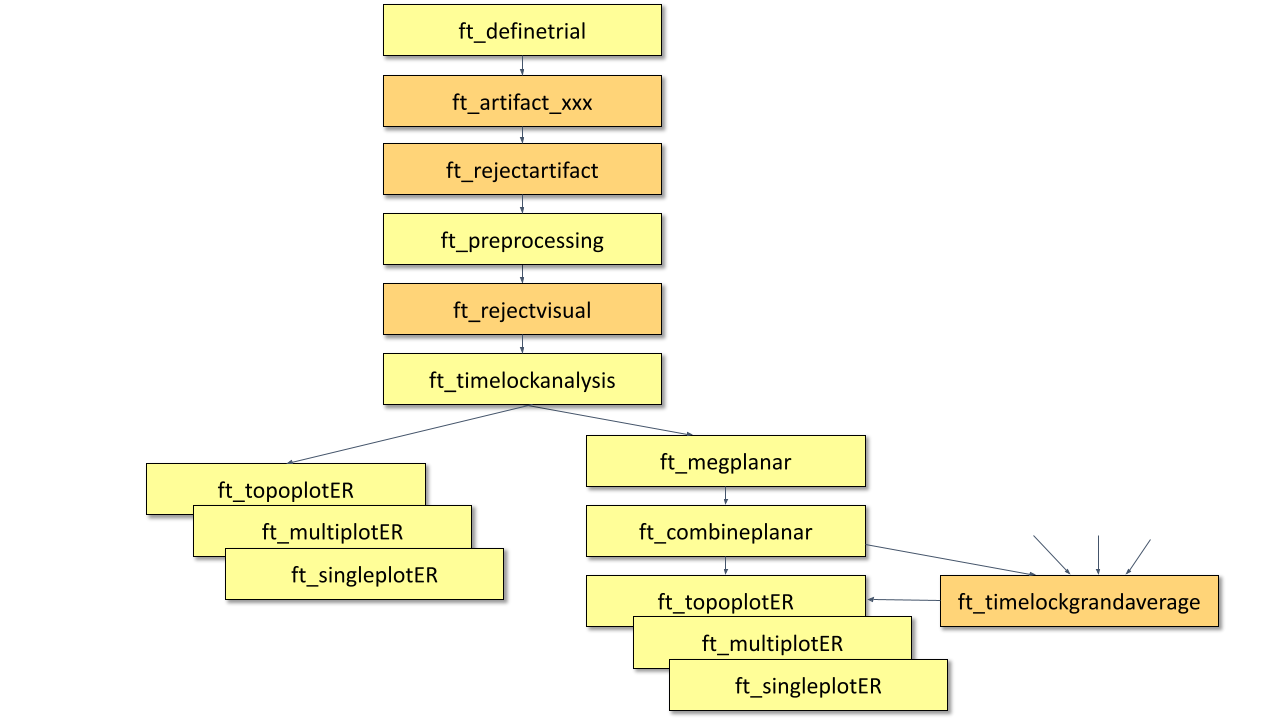Figure: A schematic overview of the steps in averaging of event-related fields.

## Preprocessing

### Reading in the data

We will now read and preprocess the data. If you would like to continue directly with the already preprocessed data, you can download dataFIC_LP.mat, dataFC_LP.mat and dataIC_LP.mat. Load the data into MATLAB with the command `load` and skip to Timelockanalysis.

Otherwise run the following code:

The ft_definetrial and ft_preprocessing functions require the original MEG dataset, which is available here

Do the trial definition for the all conditions together:

``````cfg                         = [];
cfg.dataset                 = 'Subject01.ds';
cfg.trialfun                = 'ft_trialfun_general'; % this is the default
cfg.trialdef.eventtype      = 'backpanel trigger';
cfg.trialdef.eventvalue     = [3 5 9]; % the values of the stimulus trigger for the three conditions
% 3 = fully incongruent (FIC), 5 = initially congruent (IC), 9 = fully congruent (FC)
cfg.trialdef.prestim        = 1; % in seconds
cfg.trialdef.poststim       = 2; % in seconds

cfg = ft_definetrial(cfg);
``````

### Cleaning

``````% remove the trials that have artifacts from the trl
cfg.trl([2, 5, 6, 8, 9, 10, 12, 39, 43, 46, 49, 52, 58, 84, 102, 107, 114, 115, 116, 119, 121, 123, 126, 127, 128, 133, 137, 143, 144, 147, 149, 158, 181, 229, 230, 233, 241, 243, 245, 250, 254, 260],:) = [];

% preprocess the data
cfg.channel    = {'MEG', '-MLP31', '-MLO12'};        % read all MEG channels except MLP31 and MLO12
cfg.demean     = 'yes';
cfg.baselinewindow  = [-0.2 0];
cfg.lpfilter   = 'yes';                              % apply lowpass filter
cfg.lpfreq     = 35;                                 % lowpass at 35 Hz.

data_all = ft_preprocessing(cfg);
``````

These data have been cleaned from artifacts by removing several trials and two sensors; see the visual artifact rejection tutorial.

A note about padding: The padding parameter (cfg.padding) defines the duration to which the data in the trial will be padded (i.e. data-padded, not zero-padded). The padding is removed from the trial after filtering. Padding the data is beneficial, since the edge artifacts that are typically seen after filtering will be in the padding and not in the part of interest. Padding can also be relevant for DFT filtering of the 50Hz line noise artifact: long padding ensures a higher frequency resolution for the DFT filter, causing a narrower notch to be removed from the data. Padding can only be done on data that is stored in continuous format, therefore it is not used here.

For subsequent analysis we split the data into three different data structures, one for each condition (fully incongruent condition FIC, fully congruent condition FC, and initially congruent IC).

``````cfg = [];
cfg.trials = data_all.trialinfo == 3;
dataFIC_LP = ft_selectdata(cfg, data_all);

cfg = [];
cfg.trials = data_all.trialinfo == 5;
dataIC_LP = ft_selectdata(cfg, data_all);

cfg = [];
cfg.trials = data_all.trialinfo == 9;
dataFC_LP = ft_selectdata(cfg, data_all);
``````

Subsequently you can save the data to disk.

``````  save dataFIC_LP dataFIC_LP
save dataFC_LP dataFC_LP
save dataIC_LP dataIC_LP
``````

If preprocessing was done as described, the data structure will have the following fields:

``````dataFIC_LP =
hdr: [1x1 struct]
label: {149x1 cell}
time: {1x77 cell}
trial: {1x77 cell}
fsample: 300
sampleinfo: [77x2 double]
trialinfo: [77x1 double]
elec: [1x1 struct]
cfg: [1x1 struct]
``````

Note that ‘dataFIC_LP.label’ has 149 in stead of 151 labels since channels MLP31 and MLO12 were excluded. ‘dataFIC-LP.trial’ has 76 in stead of 87 trials because 10 trials were rejected because of artifacts.

The most important fields are ‘dataFIC_LP.trial’ containing the individual trials and ‘data.time’ containing the time vector for each trial. To visualize the single trial data (trial 1) of a single channel (channel 130) you can do the following:

``````plot(dataFIC_LP.time{1}, dataFIC_LP.trial{1}(130,:))
``````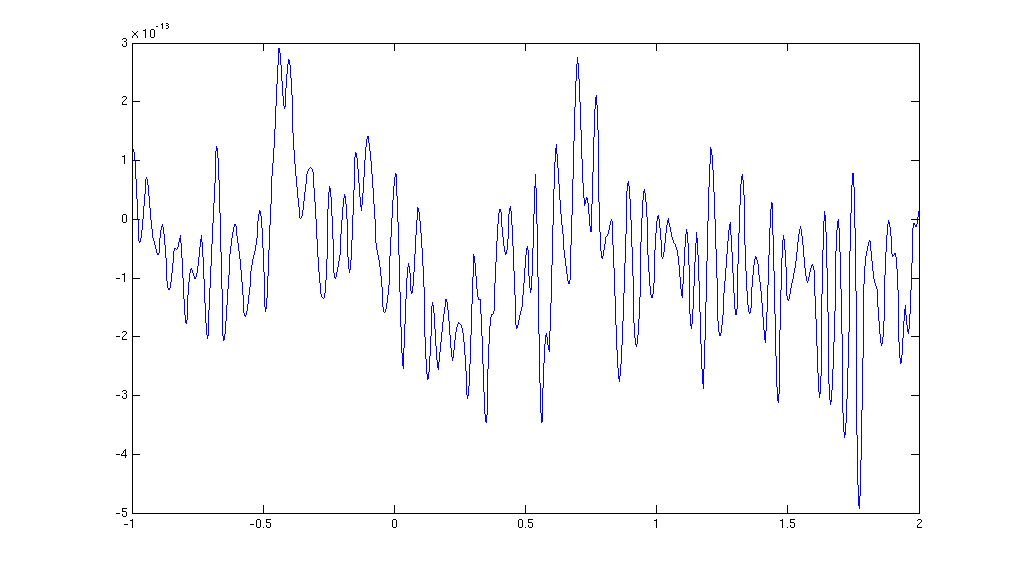Figure: The MEG data from a single trial in a single sensor obtained after ft_preprocessing.

## Timelockanalysis

The function ft_timelockanalysis makes averages of all the trials in a data structure. It requires preprocessed data (see above), which is available from dataFIC_LP.mat, dataFC_LP.mat and dataIC_LP.mat.

``````load dataFIC_LP
``````

The trials belonging to one condition will now be averaged with the onset of the stimulus time aligned to the zero-time point (the onset of the last word in the sentence). This is done with the function ft_timelockanalysis. The input to this procedure is the dataFIC_LP structure generated by ft_preprocessing. No special settings are necessary here. Thus specify an empty configuration.

``````cfg = [];
avgFIC = ft_timelockanalysis(cfg, dataFIC_LP);
avgFC = ft_timelockanalysis(cfg, dataFC_LP);
avgIC = ft_timelockanalysis(cfg, dataIC_LP);
``````

The output is the data structure avgFIC with the following fields:

``````avgFIC =
time: [-1 -0.9967 -0.9933 -0.9900 -0.9867 … ]
label: {149x1 cell}
elec: [1x1 struct]
avg: [149x900 double]
var: [149x900 double]
dof: [149x900 double]
dimord: 'chan_time'
cfg: [1x1 struct]
``````

The most important field is avgFIC.avg, containing the average over all trials for each sensor.

## Plot the results (axial gradients)

Using the plot functions ft_multiplotER, ft_singleplotER and ft_topoplotER you can make plots of the average. You can find information about plotting also in the Plotting data at the channel and source level tutorial.

Use ft_multiplotER to plot all sensors in one figure:

``````cfg = [];
cfg.showlabels = 'yes';
cfg.fontsize = 6;
cfg.layout = 'CTF151_helmet.mat';
cfg.ylim = [-3e-13 3e-13];
ft_multiplotER(cfg, avgFIC);
``````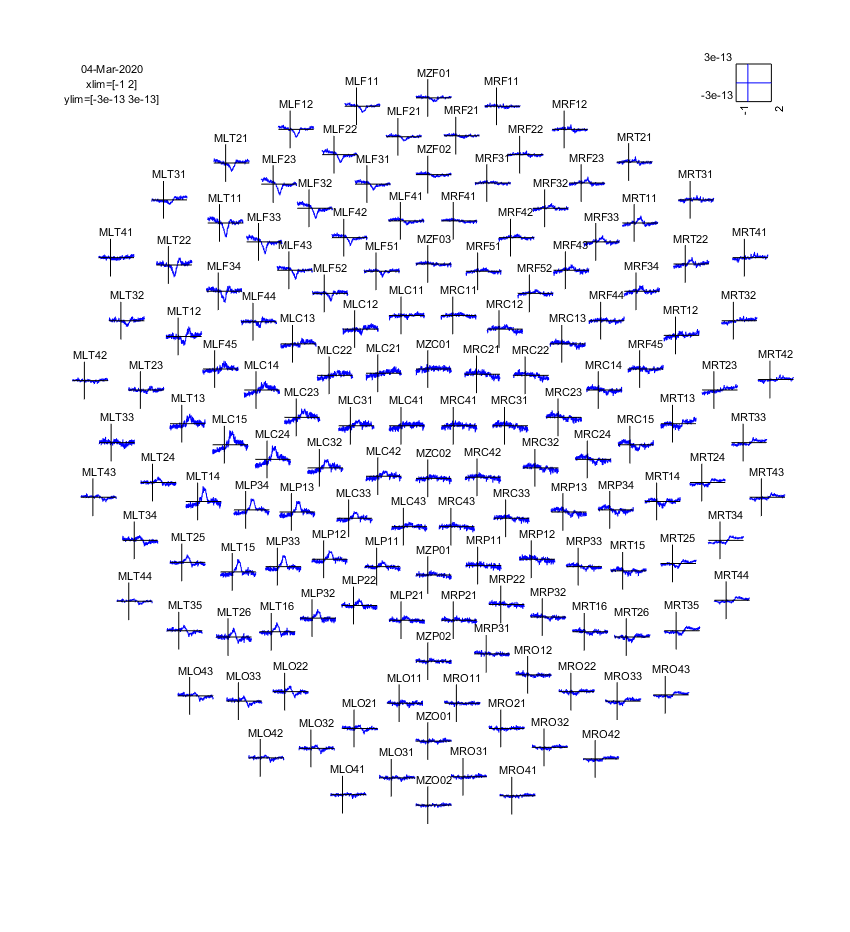Figure: The event-related fields plotted using ft_multiplotER. The event-related fields were calculated using ft_preprocessing followed by ft_timelockanalysis.

This plots the event-related fields for all sensors arranged topographically according to their position in the helmet. You can use the zoom button (magnifying glass) to enlarge parts of the figure. You can use multiple data arguments in the input, and these will be displayed together:

``````cfg = [];
cfg.showlabels = 'no';
cfg.fontsize = 6;
cfg.layout = 'CTF151_helmet.mat';
cfg.baseline = [-0.2 0];
cfg.xlim = [-0.2 1.0];
cfg.ylim = [-3e-13 3e-13];
ft_multiplotER(cfg, avgFC, avgIC, avgFIC);
``````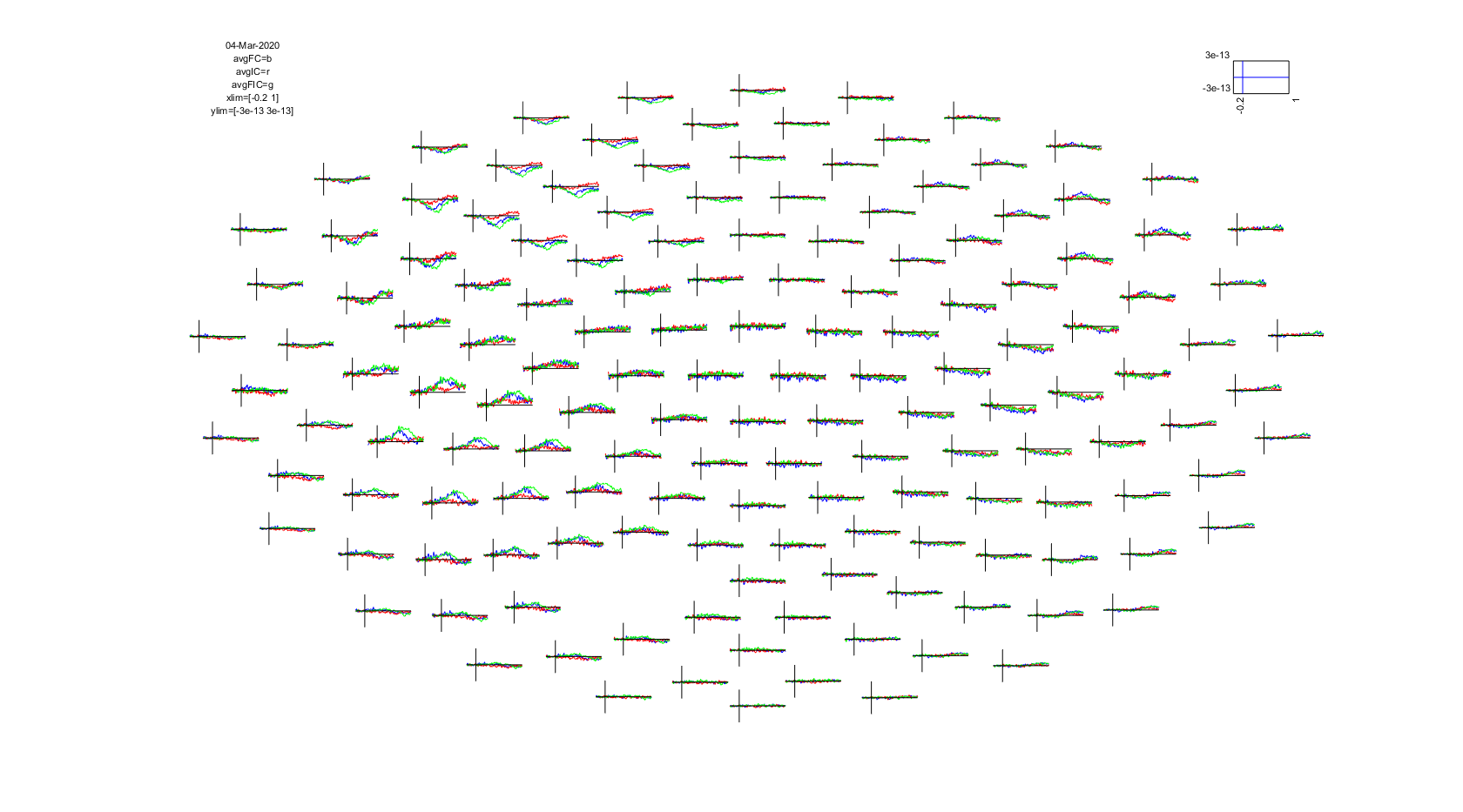Figure: The event-related fields for three conditions plotted simultaneously using ft_multiplotER.

To plot the data of a single sensor you can use ft_singleplotER while specifying the name of the channel you are interested in, for instance MLC24:

``````cfg = [];
cfg.xlim = [-0.2 1.0];
cfg.ylim = [-1e-13 3e-13];
cfg.channel = 'MLC24';
ft_singleplotER(cfg, avgFC, avgIC, avgFIC);
``````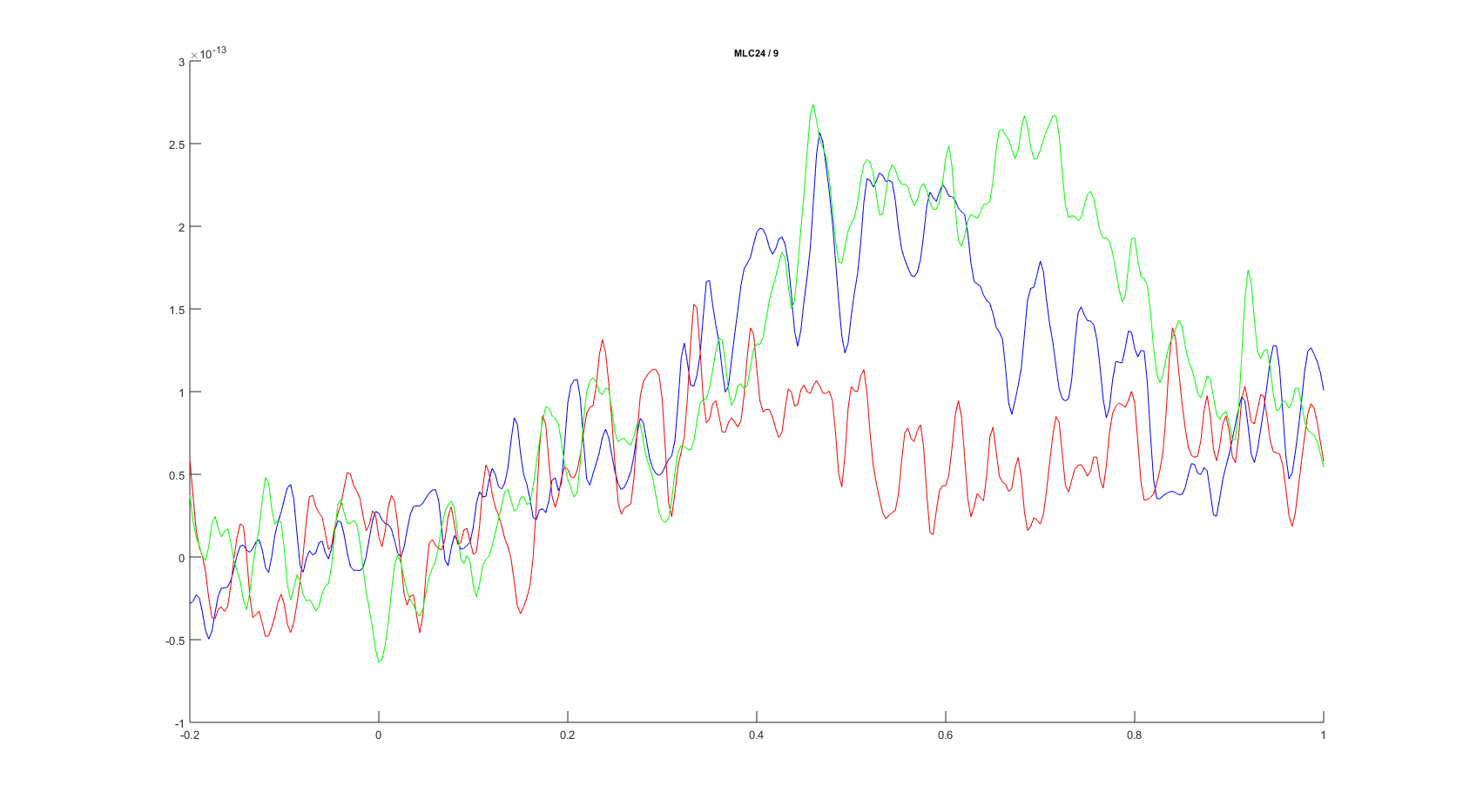Figure: The event-related fields plotted for three conditions for sensor MLC24 using ft_singleplotER.

To plot the topographic distribution of the data averaged over the time interval from 0.3 to 0.5 seconds you can use the following command:

``````cfg = [];
cfg.xlim = [0.3 0.5];
cfg.colorbar = 'yes';
cfg.layout = 'CTF151_helmet.mat';
ft_topoplotER(cfg, avgFIC);
``````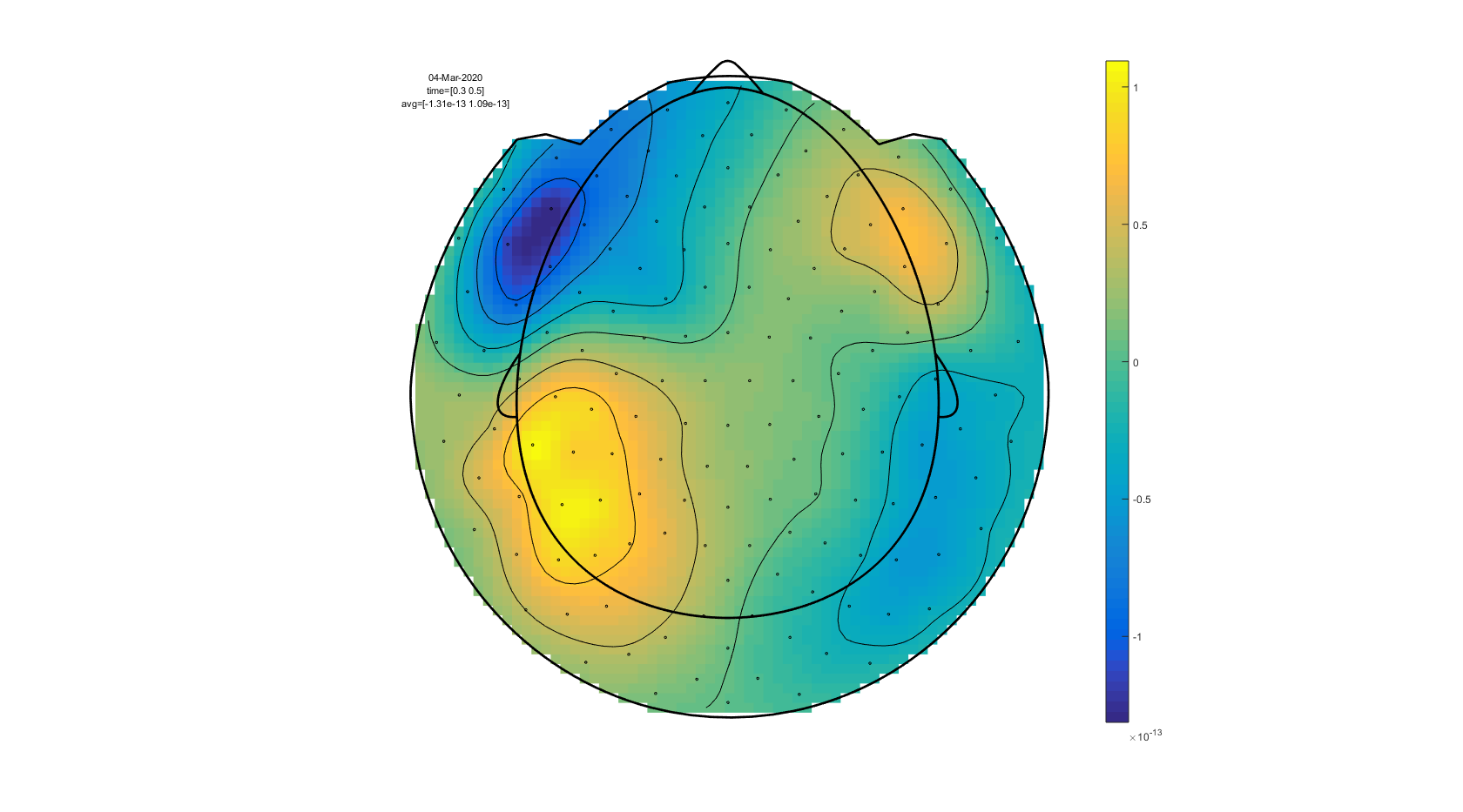Figure: A topographic plot of the event-related fields obtained using ft_topoplotER.

To plot a sequence of topographic plots you can define cfg.xlim to be a vector:

``````cfg        = [];
cfg.xlim   = [-0.2 : 0.1 : 1.0];  % Define 12 time intervals
cfg.zlim   = [-2e-13 2e-13];      % Set the 'color' limits.
cfg.layout = 'CTF151_helmet.mat';
ft_topoplotER(cfg, avgFIC);
``````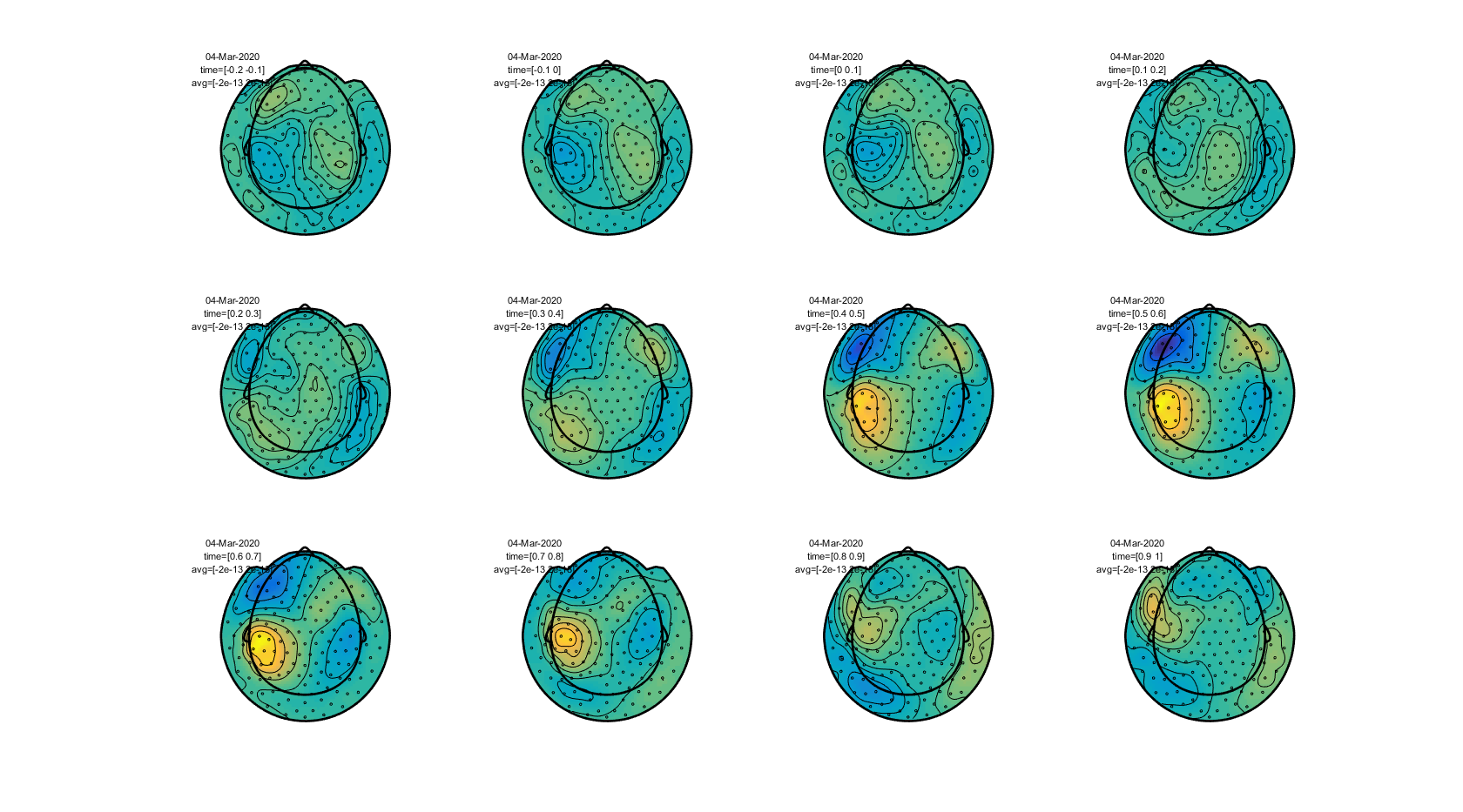Figure: The topography of event-related fields over time obtained using ft_topoplotER.

#### Exercise 1

What changes in the data if you extend the baseline correction (which is initially from -200 ms to 0 ms) to a longer period from -500 ms to 0?

Apply a band-pass filter in the preprocessing instead of only a low-pass filter. Use for example the values from 1 Hz to 30 Hz. What changes in the data? What are the pros and cons of using a high-pass filter?

#### Exercise 2

Which type of source configuration can explain the topography?

## Calculate the planar gradient

With ft_megplanar we calculate the planar gradient of the averaged data. Ft_megplanar is used to compute the gradient of the magnetic field that is tangential to the head surface. This leads to a 2-dimensional signal at each of the sensor locations, which are denoted as ‘horizontal’ and ‘vertical’ components of the planar gradient. This terminology is based on the analogy with a 2-dimensional Cartesian coordinate system, with a horizontal and vertical axis: the gradient axes are not ‘horizontal’ and ‘vertical’ w.r.t. the surroundings.

The planar gradient at a given sensor location can be approximated by comparing the field at that sensor with its neighbors (i.e. by means of a finite difference estimate of the derivative). To compute the magnitude of the gradient, the two orthogonal gradients on a single sensor location can be combined using Pythagoras rule with the FieldTrip function ft_combineplanar.

Calculate the planar gradient of the averaged data:

``````cfg                 = [];
cfg.feedback        = 'yes';
cfg.method          = 'template';
cfg.neighbours      = ft_prepare_neighbours(cfg, avgFIC);

cfg.planarmethod    = 'sincos';
avgFICplanar        = ft_megplanar(cfg, avgFIC);
``````

Compute the magnitude of the planar gradient by combining the horizontal and vertical components of the planar gradient according to Pythagoras rule:

``````cfg = [];
avgFICplanarComb = ft_combineplanar(cfg, avgFICplanar);
``````

## Plot the results (planar gradients)

To compare the axial gradient data to the planar gradient data we plot them both in one figure here.

Plot the results of the field of the axial gradiometers and the planar gradient to compare the topographies:

``````cfg = [];
cfg.xlim = [0.3 0.5];
cfg.zlim = 'maxmin';
cfg.colorbar = 'yes';
cfg.layout = 'CTF151_helmet.mat';
cfg.figure  = subplot(121);
ft_topoplotER(cfg, avgFIC)

colorbar; % you can also try out cfg.colorbar = 'south'

cfg.zlim = 'maxabs';
cfg.layout = 'CTF151_helmet.mat';
cfg.figure  = subplot(122);
ft_topoplotER(cfg, avgFICplanarComb);
``````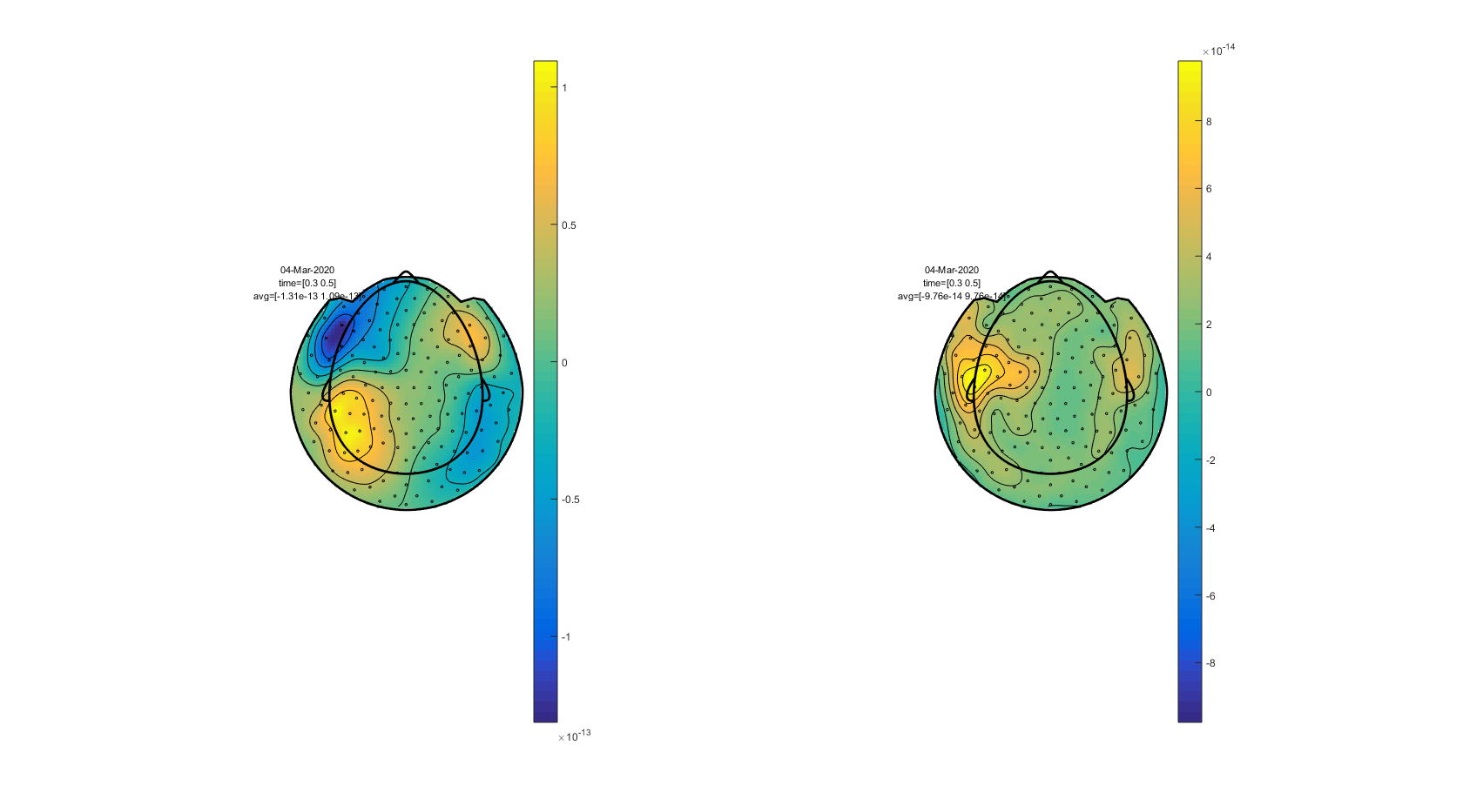Figure: A comparison of event-related fields from the axial gradiometers (left) and the planar gradient (right). The planar gradient was calculated using ft_megplanar and ft_combineplanar.

#### Exercise 3

Compare the axial and planar gradient field

Why are there only positive values above the sources in the representation of the combined planar gradient?

Explain the topography of the planar gradient from the fields of the axial gradient

## Grand average over subjects

Finally you can make a grand-average over all our four subjects with ft_timelockgrandaverage. Before calculating the grand average, the data of each subject can be realigned to standard sensor positions with ft_megrealign. For this step, there are the additional datasets Subject02.zip, Subject03.zip, and Subject04.zip.

``````help ft_timelockgrandaverage